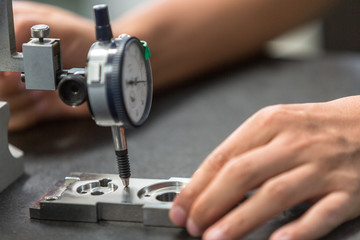# Wow! A Device Invented to Measure Voltage! Check It Out!379How does electricity flow in a conductor? It is because of the potential difference across the conductor. How is this potential difference measured? It is measured with a device called voltmeter.

### INTRODUCTION ?

How does electricity flow in a conductor? It is because of the potential difference across the conductor. How is this potential difference measured? It is measured with a device called voltmeter.

### DEFINITION ?

Voltmeter is used to measure the voltage or difference in electric potential between two points in a circuit. It has many practical applications. Voltmeter is used to determine if the battery is charged or not. When a car does not start, people generally check the battery voltage to determine if this is the issue or not.

### DIFFERENCE BETWEEN CURRENT AND VOLTAGE ?

Voltage is the electrical potential difference per unit charge, or can be defined as the energy contained within an electrical circuit. Current is the rate at which electric charges passes through the circuit. Some voltmeters are designed to measure direct current (DC), some are specially designed for measuring alternating current (AC) and some are designed to measure Radio frequency (RF) voltage.

### ANALOG VOLTMETER ?

An analog voltmeter is the one which has a scale which moves in proportion to the current?s voltage. Higher the current higher the scale moves. Any measurement that is convertible to voltage can be measured on analog voltmeter that is accurately calibrated. The examples of such measurements are pressure, temperature, liquid flow (viscosity). A basic analog voltmeter consists of a galvanometer in series with a high resistance. The combination of accuracy of galvanometer and the resistance, determine the range of voltages that can be measured by the voltmeter.

### DIGITAL VOLTMETER ?

A digital voltmeter is one which shows the numerical value of the current?s voltage. Practical laboratories have digital voltmeters which range from one thousand to three thousand volts. Commercially used voltmeters have multiple ranges, increasing in powers of 10. Like 0-10 Volts, 0-100 Volts.

### USAGE OF VOLTMETER?

It is of utmost importance that the electrical connections in the circuit are correct; else the values read by the measuring instruments can be often incorrect. The rule of current says ?

### V = I / R (V = voltage, I = current, R = resistance)

The voltmeter offers high resistance. If the resistance is low, then it will withdraw a larger current (lower the resistance higher the current). As such, the circuit under consideration would not receive the proper amount of current. Therefore, voltmeter would give incorrect readings then. Therefore, voltmeter is designed to have high resistance. It is always connected in parallel to the circuit.